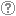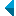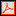# Search the repositoryQuery: search in TitleAuthorAbstractKeywordsFull textYear of publishing ANDORAND NOT search in TitleAuthorAbstractKeywordsFull textYear of publishing ANDORAND NOT search in TitleAuthorAbstractKeywordsFull textYear of publishing ANDORAND NOT search in TitleAuthorAbstractKeywordsFull textYear of publishing Work type: All work types Habilitation (m4) Specialist thesis (m3) High school thesis (m6) Bachelor work * (dip) Master disertations * (mag) Doctorate disertations * (dok) Research Data or Corpuses (data) * old and bologna study programme Language: All languagesSlovenianEnglishGermanCroatianSerbianAfrikaansAlbanianArabicArmenianAzerbaijaniBosnianBulgarianCatalanChineseCzechDanishDutchEstonianFinnishFrenchGeorgianGerman (Austria)GreekHungarianIrishItalianJapaneseKazakhKoreanLatinLatvianLithuanianMacedonianMalteseMontenegrinNorwegianPolishPortugueseRomanianRussianSerbian (cyrillic)Slavic languagesSlovakSpanishSwedishTurkishUkrainianMultilingualUndeterminedMiscellaneous (other)Unknown Search in: RUP    FAMNIT - Faculty of Mathematics, Science and Information Technologies    FHŠ - Faculty of Humanities    FM - Faculty of Management    FTŠ Turistica - Turistica – College of Tourism Portorož    FVZ - Faculty of Health Sciences    IAM - Andrej Marušič Institute    PEF - Faculty of Education    UPR - University of Primorska    ZUP - University of Primorska PressCOBISS    University of Primorska, University Library - all departments Options: Show only hits with full text Reset

 1 - 10 / 12121.O nekaterih problemih, ki so povezani s Terwilligerjevimi algebrami in razdaljno-uravnoteženimi grafi : doktorska disertacijaBlas Fernández, 2023, doctoral dissertation 2.On bipartite graphs with exactly one irreducible T-module with endpoint 1, which is thinBlas Fernández, Štefko Miklavič, 2021, original scientific articleKeywords: distance-biregular graph, Terwilliger algebra, irreducible modulePublished in RUP: 18.10.2021; Views: 765; Downloads: 27Link to full text 3.A combinatorial basis for Terwilliger algebra modules of a bipartite distance-regular graphMark MacLean, Safet Penjić, 2021, original scientific articleKeywords: bipartite distance-regular graph, Terwilliger algebra, subconstituent algebra, T-modules of endpoint 2Published in RUP: 06.05.2021; Views: 607; Downloads: 29Link to full text 4.On the Terwilliger algebra of a certain family of bipartite distance-regular graphs with Delta_2 = 0Štefko Miklavič, Safet Penjić, 2020, original scientific articleKeywords: distance-regular graphs, Terwilliger algebra, irreducible modulesPublished in RUP: 17.08.2020; Views: 1144; Downloads: 39Full text (420,51 KB) 5.On a certain class of 1-thin distance-regular graphsMark MacLean, Štefko Miklavič, 2020, original scientific articleKeywords: distance-regular graph, Terwilliger algebra, subconstituent algebraPublished in RUP: 20.07.2020; Views: 1178; Downloads: 85Full text (379,60 KB) 6.O Terwilligerjevi algebri dvodelnih razdaljno-regularnih grafov : doktorska disertacijaSafet Penjić, 2019, doctoral dissertationKeywords: bipartite distance-regular graph, Terwilliger algebra, subconstituent algebra, Q-polynomial property, equitable partitionPublished in RUP: 04.06.2019; Views: 2080; Downloads: 30Link to full text 7.On the Terwilliger algebra of bipartite distance-regular graphs with $G_{i-1,i-1,1}(x, y, z) = alpha_i + beta_i G_{1,1,i%1}(x, y, z)$ : 31th Ljubljana - Leoben Graph Theory Seminar, September 3-4, 2018, LoebenSafet Penjić, 2018, unpublished conference contributionKeywords: distance-regular graphs, Terwilliger algebra, irreducible modulesPublished in RUP: 17.09.2018; Views: 1507; Downloads: 24Link to full text 8.An A-invariant subspace for bipartite distance-regular graphs with exactly two irreducible T-modules with endpoint 2, both thinMark MacLean, Štefko Miklavič, Safet Penjić, 2018, original scientific articleKeywords: distance-regular graph, Terwilliger algebra, subconstituent algebra, A-invariant subspacePublished in RUP: 02.03.2018; Views: 1861; Downloads: 82Link to full text 9.On the Terwilliger algebra of bipartite distance-regular graphs with [Delta][sub]2 = 0 and c[sub]2=1Mark MacLean, Štefko Miklavič, Safet Penjić, 2016, original scientific articleAbstract: Let ▫$\Gamma$▫ denote a bipartite distance-regular graph with diameter ▫$D \geq 4$▫ and valency ▫$k \geq 3$▫. Let ▫$X$▫ denote the vertex set of ▫$\Gamma$▫, and let ▫$A$▫ denote the adjacency matrix of ▫$\Gamma$▫. For ▫$x \in X$▫ and for ▫$0 \leq i \leq D$▫, let ▫$\operatorname{\Gamma}_i(x)$▫ denote the set of vertices in ▫$X$▫ that are distance ▫$i$▫ from vertex ▫$x$▫. Define a parameter ▫$\operatorname{\Delta}_2$▫ in terms of the intersection numbers by ▫$\operatorname{\Delta}_2 = (k - 2)(c_3 - 1) -(c_2 - 1) p_{22}^2$▫. We first show that ▫$\operatorname{\Delta}_2 = 0$▫ implies that ▫$D \leq 5$▫ or ▫$c_2 \in \{1, 2 \}$▫. For ▫$x \in X$▫ let ▫$T = T(x)$▫ denote the subalgebra of ▫$\text{Mat}_X(\mathbb{C})$▫ generated by ▫$A, E_0^\ast, E_1^\ast, \ldots, E_D^\ast$▫, where for ▫$0 \leq i \leq D$, $E_i^\ast$▫ represents the projection onto the▫ $i$▫th subconstituent of ▫$\Gamma$▫ with respect to ▫$x$▫. We refer to ▫$T$▫ as the Terwilliger algebra of ▫$\Gamma$▫ with respect to ▫$x$▫. By the endpoint of an irreducible ▫$T$▫-module ▫$W$▫ we mean ▫$\min \{i | E_i^\ast W \ne 0 \}$▫. In this paper we assume ▫$\Gamma$▫ has the property that for ▫$2 \leq i \leq D - 1$▫, there exist complex scalars ▫$\alpha_i$▫, ▫$\beta_i$▫ such that for all ▫$x, y, z \in X$▫ with ▫$\partial(x, y) = 2$▫, ▫$\partial(x, z) = i$▫, ▫$\partial(y, z) = i$▫, we have ▫$\alpha_i + \beta_i | \operatorname{\Gamma}_1(x) \cap \operatorname{\Gamma}_1(y) \cap \operatorname{\Gamma}_{i - 1}(z) | = | \operatorname{\Gamma}_{i - 1}(x) \cap \operatorname{\Gamma}_{i - 1}(y) \cap \operatorname{\Gamma}_1(z) |$▫. We additionally assume that▫ $\operatorname{\Delta}_2 = 0$▫ with ▫$c_2 = 1$▫. Under the above assumptions we study the algebra ▫$T$▫. We show that if ▫$\Gamma$▫ is not almost 2-homogeneous, then up to isomorphism there exists exactly one irreducible ▫$T$▫-module with endpoint 2. We give an orthogonal basis for this ▫$T$▫-module, and we give the action of ▫$A$▫ on this basis.Keywords: distance-regular graphs, terwilliger algebra, subconstituent algebraPublished in RUP: 14.11.2017; Views: 2024; Downloads: 141Link to full text 10.Leonard triples and hypercubesŠtefko Miklavič, 2007, original scientific articleAbstract: Let ▫$V$▫ denote a vector space over ▫$\mathbb{C}$▫ with finite positive dimension. By a Leonard triple on ▫$V$▫ we mean an ordered triple of linear operators on ▫$V$▫ such that for each of these operators there exists a basis of ▫$V$▫ with respect to which the matrix representing that operator is diagonal and the matrices representing the other two operators are irreducible tridiagonal. Let ▫$D$▫ denote a positive integer and let ▫${\mathcal{Q}}_D$▫ denote the graph of the ▫$D$▫-dimensional hypercube. Let ▫$X$ denote the vertex set of ▫${\mathcal{Q}}_D$▫ and let ▫$A \in {\mathrm{Mat}}_X ({\mathbb{C}})$▫ denote the adjacency matrix of ▫${\mathcal{Q}}_D$▫. Fix ▫$x \in X$▫ and let ▫$A^\ast \in {\mathrm{Mat}}_X({\mathbb{C}})$▫ denote the corresponding dual adjacency matrix. Let ▫$T$▫ denote the subalgebra of ▫${\mathrm{Mat}}_X({\mathbb{C}})$ generated by ▫$A,A^\ast$▫. We refer to ▫$T$▫ as the Terwilliger algebra of ▫${\mathcal{Q}}_D$▫ with respect to ▫$x$▫. The matrices ▫$A$▫ and ▫$A^\ast$▫ are related by the fact that ▫$2iA = A^\ast A^\varepsilon - A^\varepsilon A^\ast$▫ and ▫$2iA^\ast = A^\varepsilon A - AA^\varepsilon$▫, where ▫$2iA^\varepsilon = AA^\ast - A^\ast A$▫ and ▫$i^2 = -1$▫. We show that the triple ▫$A$▫, ▫$A^\ast$▫, ▫$A^\varepsilon$▫ acts on each irreducible ▫$T$▫-module as a Leonard triple. We give a detailed description of these Leonard triples.Keywords: mathematics, graph theory, Leonard triple, distance-regular graph, hypercube, Terwilliger algebraPublished in RUP: 15.10.2013; Views: 3370; Downloads: 119Link to full text
Search done in 0 sec.